## A block of mass 5.6 kg is attached to a horizontal spring on a rough floor. Initially the spring is compressed 3.5 cm. The spring has a forc

Question

A block of mass 5.6 kg is attached to a horizontal spring on a rough floor. Initially the spring is compressed 3.5 cm. The spring has a force constant of 1040 N/m. The coefficient of kinetic friction between the block and the floor is 0.26. The block is released from rest. The speed of the block after it has traveled 0.020 m is

in progress 0
6 months 2021-07-29T10:07:30+00:00 1 Answers 11 views 0

The velocity of block = 0.188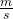Explanation:

Mass m = 5.6 kg

k = 1040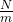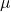= 0.26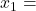0.035 m  ,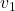= 0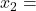0.02 m

From work energy theorem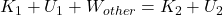——— (1)

Kinetic energy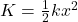——- (1)

Potential energy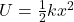——- (2)

Work done

W = F.s —— (3)

From Newton’s second law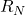= mg= 5.6 × 9.81 = 54.9 N

Friction  force = 0.4 × 54.9 = 21.9 N

Now the work done by the friction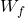= – 21.9 × 0.015= – 0.329 J

Now kinetic energy

At point 1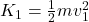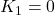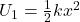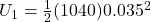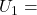0.637 J

At point 2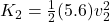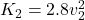Potential energy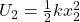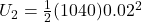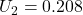J

From equation (1) we get

0 + 0.637 – 0.329 = 2.8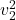+ 0.208

2.8= 0.1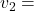0.188This is the velocity of block.